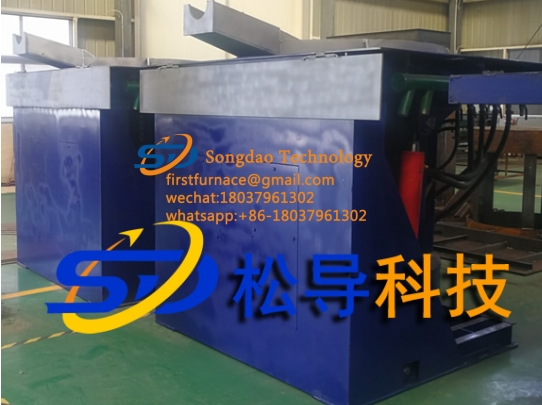### Maintenance method of induction melting furnace

Maintenance method of induction melting furnace

1. When the induction melting furnace fails, it is necessary to observe whether the parameters of the instrument of the induction melting furnace are correct when it is running, and whether there is heating, redness, loose screws and other appearance phenomena in the induction melting furnace. Whether the relationship between the intermediate frequency voltage, DC voltage, and DC current of the induction melting furnace meter is operating normally. The product of DC voltage and DC current is the intermediate frequency power, so that we can judge whether the power of the induction melting furnace is completely normal; whether the ratio of incoming line voltage, DC voltage and intermediate frequency voltage is correct. For example: 500kw induction melting furnace, the incoming line voltage is 380V, then the maximum DC voltage is 513V, and the DC current is 1000A. If the DC voltage reaches 500V and the DC current value is 1000A during operation, the operating power is normal. The ratio of DC voltage and intermediate frequency voltage can reflect the working state of the inverter. For example, if the DC voltage is 510V and the intermediate frequency voltage is 700V, the lead angle of the inverter is 36°. We use 700V/510V=1.37 to see that, in general, the ratio of intermediate frequency voltage and DC voltage is between 1.2 and 1.5, and we all think that the inverter is working normally. If the ratio is less than 1.2, the lead angle is too small, and the inverter is difficult to commutate; if it is greater than 1.5 times, the lead angle is too large, and the equipment may fail.

2. Whether the sound of the induction melting furnace is normal during operation, whether there is noise in the sound of the induction melting furnace, whether the sound is continuous, whether there is a dull reactor vibration sound and whether there is a crackling sound of ignition, etc. In short, it is different from the usual sound. to determine the sound position.

3. Ask the operator of the induction melting furnace about the status of the induction melting furnace when it breaks down. When understanding it, try to be as detailed as possible. At the same time, you should also understand the operating status of the induction melting furnace before the failure.

4. When the induction melting furnace fails, you should learn to use test instruments such as oscilloscopes and multimeters to measure the waveform, voltage, time, angle, resistance and other parameters of each point to determine the cause of the failure.

5. If the fault of the induction melting furnace is found and repaired, do not run the equipment directly after finding the fault point without any inspection, because there are often other deeper reasons behind the fault point to cause such faults.